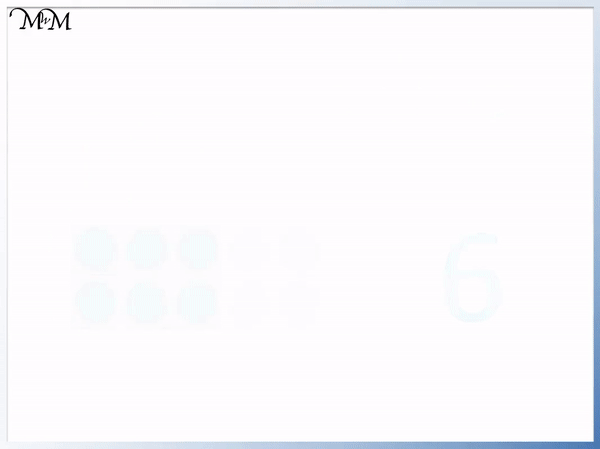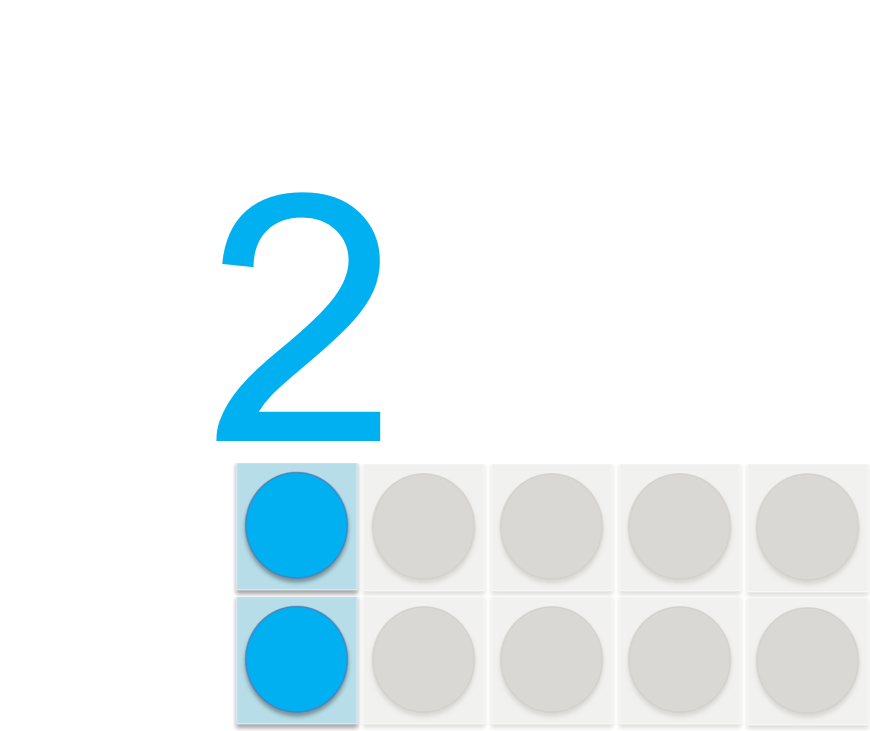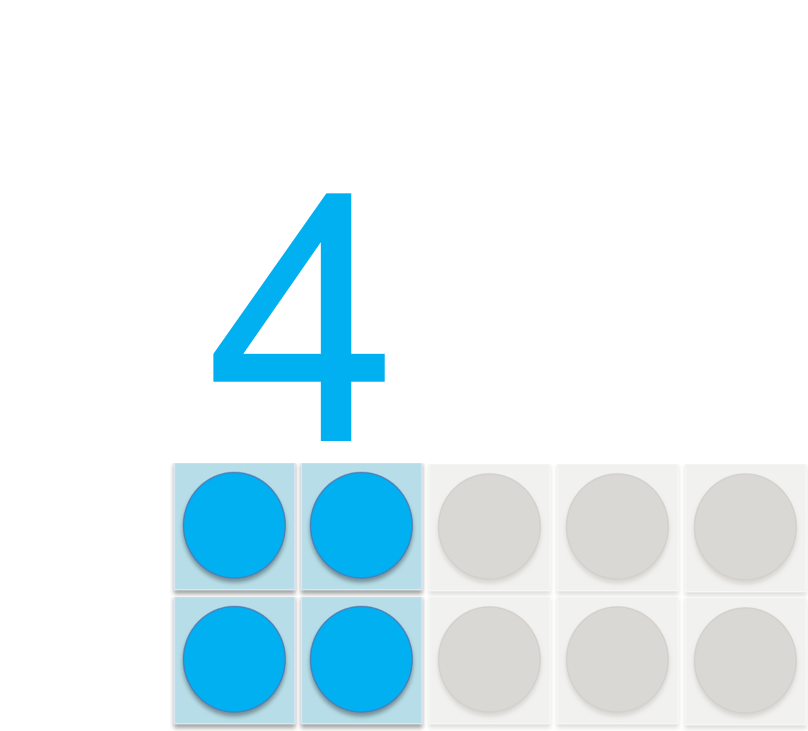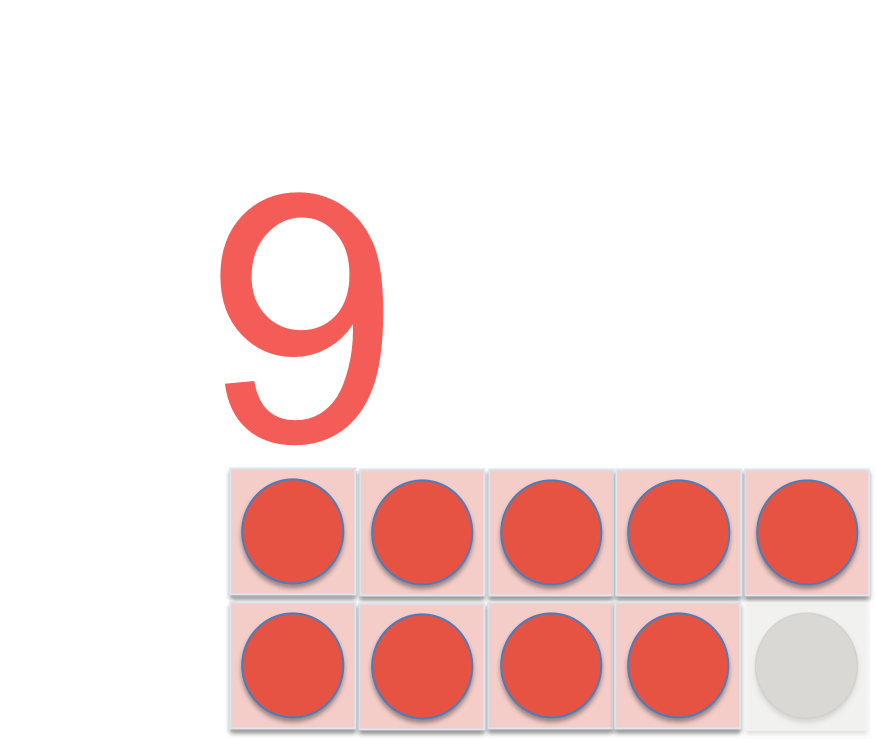# What are Even and Odd Numbers?

What are Even and Odd Numbers?• An even number is a in the two times table.
• An even number can be halved to leave another whole number.
• An odd number is not in the two times table and cannot be divided equally into two whole numbers.
• 6 can be divided into two lots of three, so it is an even number.
• 7 cannot be divided into two equal whole numbers, so it is an odd number.
• Even numbers end in 0, 2, 4, 6 or 8.
• Odd numbers end in 1, 3, 5, 7 or 9.
An even number is in the two times table, ending in 0, 2, 4, 6 or 8.

An even number is a whole number that can be halved to get another whole number.• Even numbers are in the two times table and can be arranged in pairs of .
• Odd numbers cannot be arranged in a pair and will always have a spare unit.
• We can see that the first odd number is one because it does not form a pair.
• We alternate between odd and even every time we add one.
• The first odd numbers are: 1,3, 5, 7 and 9.
• The first even numbers are: 0, 2, 4, 6, and 8.# What are Odd and Even Numbers?

Even numbers are in the two times table.

Even numbers can be arranged in pairs. This means that they can be divided into two equal parts, or halved exactly.

The whole numbers are 0, 1, 2, 3, 4, 5, 6, 7, 8, 9, 10 … onwards.

We will look at these numbers in this lesson and we will start by looking at zero.The definition of an even number is a whole number that can be halved exactly to leave another whole number.

Half of zero is zero.

And zero is a whole number.

Therefore zero is an even number.

When teaching odd and even numbers, it can be common for a child to be unsure as to whether zero is odd or even so it is important to tell them specifically that zero is actually even.

We will now look at our next example of an even number:Two is an even number. It can be halved exactly to equal one.

2 x 1 = 2

Two is in the two times table and so it is even.Four is an even number. It can be arranged a pair. When divided into two equal parts, each part is worth 2 because 4 ÷ 2 = 2.

2 x 2 = 4

Four is in the two times table and so it is even.Six is an even number. It is in the two times table. It can be divided by two to equal three.Eight is an even number. It is in the two times table and we can see that it can be arranged in pairs.

Eight is two pairs of 4.Ten is an even number. It can be halved to form two groups of five.

We can see from our list of even numbers so far that they start at zero and there is a difference of two between each even number.

If we have an even number and we add two, we will be at another even number.

Odd numbers are not in the two times table.

Odd numbers cannot be arranged in pairs. This means that they can’t be divided into two equal parts, or halved to give a whole number.

One is an example of an odd number:One is the first odd number. It can’t be arranged in pairs.Three is an odd number. It can’t be divided into two equal parts (where each part contains a whole number).

Three is not in the two times table.Five is an odd number. It is not in the two times table.Seven is an odd number. It can’t be halved (to give a whole number).Nine is an odd number. It is not in the two times table and therefore can’t be divided into two equal parts (where each part is a whole number).

Below is a summary of the even and odd numbers from one to ten. You will notice that the numbers alternate between being even and odd.

Every time we add one we change from being odd to even or even to odd.We can think of the even numbers as being the numbers in the two times table and then the odd numbers are the numbers that are in between the even numbers.Now try our lesson on Multiples of Two on a Number Grid where we learn further about the even numbers using a number grid.error: Content is protected !!